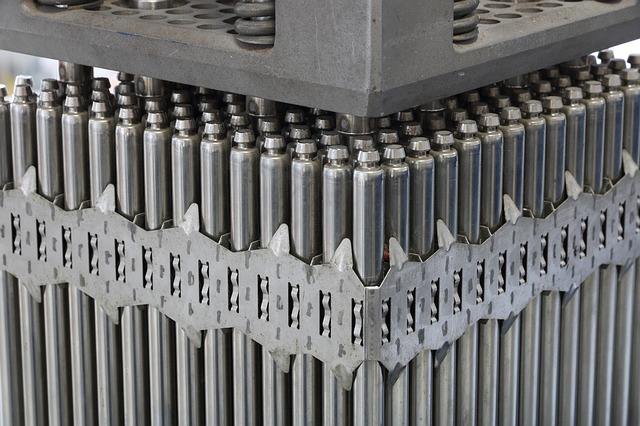Cadmium was discovered and isolated by two German chemists Karl Samuel Leberecht Hermann and Friedrich Stromeyer in 1817.

## Which element has a larger atomic radius than sulfur? Chlorine, cadmium, fluorine or oxygen?

The atomic radius of sulfur = 88 pm.

The atomic radius of Chlorine = 79 pm.

The atomic radius of fluorine = 42 pm

The atomic radius of oxygen = 48 pm

pm =  picometers ( 1×10−12 m)

Cadmium is found in ore like greenockite. Cadmium is produced as a by-product of mining. It is collected and refined from Zinc and sulfidic ores. Rocks mined for phosphate fertilizer also contain a small amount of cadmium. China is the top producer of Cadmium followed by South Korea and Japan.

## What is the number of valence electrons in cadmium (cd)?

Cadmium has two valence electrons. Its electronic configuration is 1s2, 2s2, 2p6, 3s2, 3p6, 4s2, 3d10, 4p6, 4d10, and 5s2. ([Kr] 4d10 5s2). There are 48 electrons, 48 protons and 64 neutrons.## If a sample of pure cadmium has a mass of 18.2 grams, how many moles of cadmium are in the sample?

Given data;

mass of substance = 18.2 grams.

Required data:

find the number of moles =?

Formula:

moles = mass ÷ molar mass.

As we know the molar mass of cadmium is 112.41 gram/mol.

Now put the values in the above formula.

Moles = 18.2 g ÷ 112.41g/mol = 0.161 mol.

No of moles of cadmium = 0.161 mol.